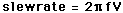# Op-amp Slew Rate

One of the practical op-amp limitations is the rate at which the output voltage can change. The limiting rate of change for a device is called its "slew rate". The slew rate for the 741 is 0.5V/microsecond compared to 100V/microsecond for a high-speed op-amp. The LH0063C has a slew rate of 6000V/microsecond. The slew-rate imposes high-frequency limitations on the device. At some critical frequency, an output swing equal to the supply voltages will require a slew rate faster than the maximum slew rate of the device. Above that frequency, the amplitude of distortion-free output voltage swing will be limited.

The slew rate is inherently limited by the small internal drive currents of an op-amp but is also limited by internal capacitance designed to compensate against high frequency oscillations. Some op-amps are externally compensated and therefore offer some control over the slew rate.

The slew rate for a given amplitude V and frequency f isFor distortion free amplitude v = V

at frequency f= x 10^Hz

a minimum slew rate of volts per microsecond is required.

Calculation notes: If the amplitude or frequency is changed, the minimum slewrate is calculated. If you wish to calculate amplitude or frequency for any value of slewrate, enter the number for slewrate and click on the active text for amplitude or frequency. If slewrate is changed, the other parameters will not be forced to be consistent until you click on one of them.

Index

Electronics concepts

Op-amp concepts

 HyperPhysics*****Electricity and magnetism R Nave
Go Back

# Op-amp Temperature Dependence

One of the practical op-amp limitations is the temperature dependence of internal parameters. Most such variations are taken care of by the negative feedback which is used in all op-amp circuits. The only temperature variations which are of practical significance are those of the the input offset voltage and current. When the offset voltage has been trimmed to zero, temperature variations can cause there to some drift of the output.

Index

Electronics concepts

Op-amp concepts

 HyperPhysics*****Electricity and magnetism R Nave
Go Back